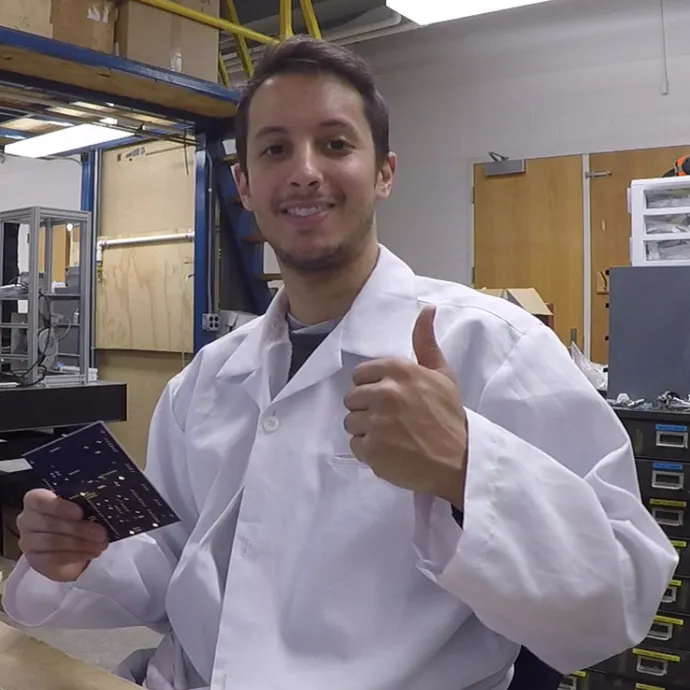Created July 11, 2018 © GPL3+

# AiRobot

AiRobot monitors and modifies the environment in your home by using a robot that receives data from a collection of networked sensors.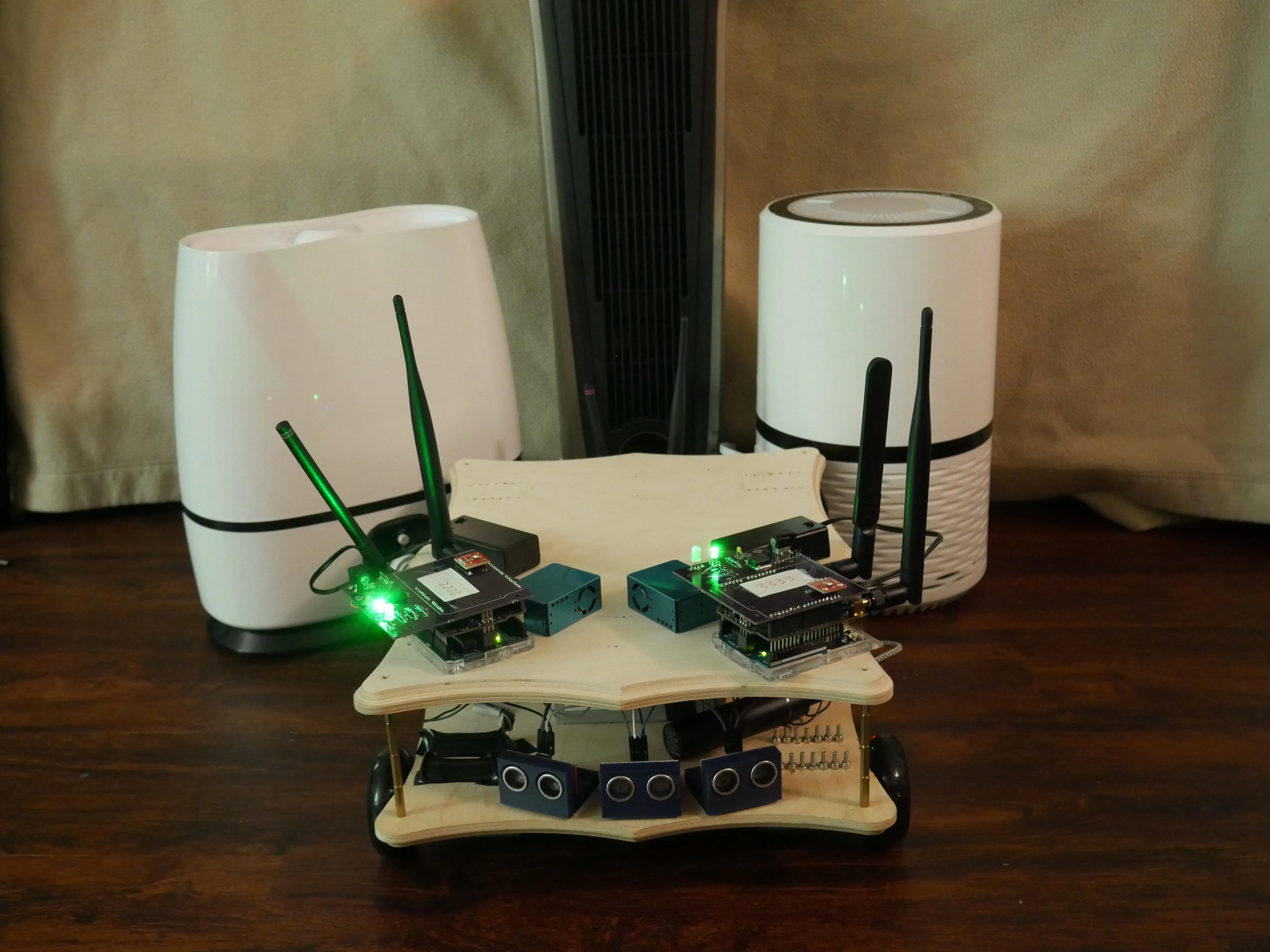## Things used in this project

### Hardware components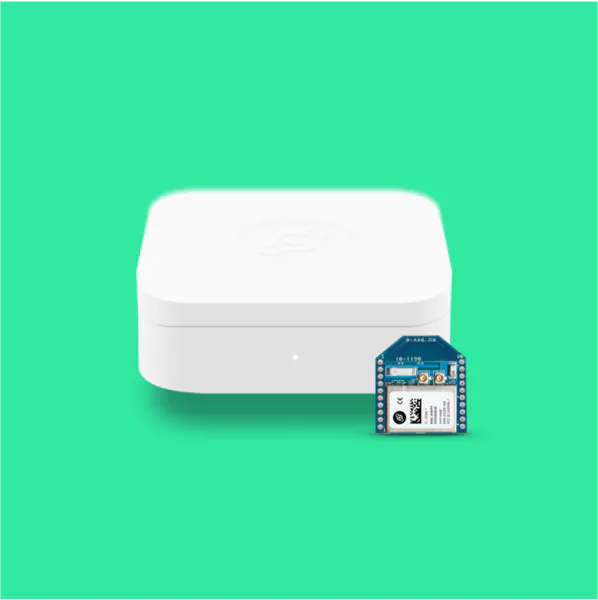Helium Starter Kit (LEGACY) Helium Ethernet kit allows us to connect to the cloud. We used the provided arduino compatible components on the AiRobot
×1
 Helium Arduino/mbed Adapter These Helium Arduino adapters were used on the Arduino Uno's for the AiRobot wall mount sensors
×2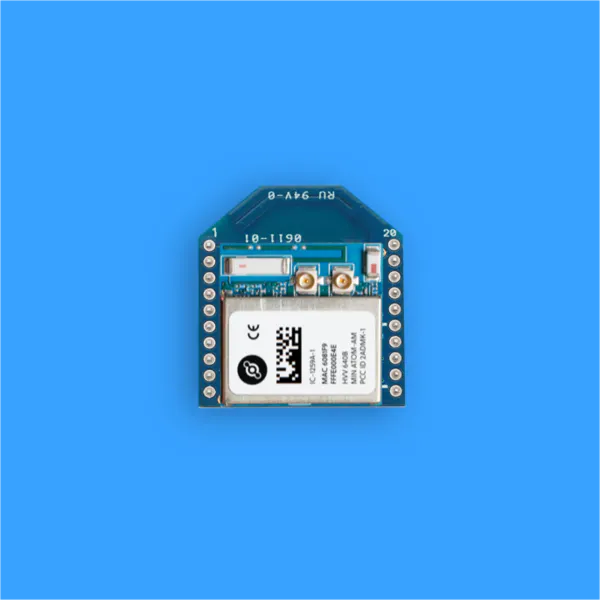Helium Atom Xbee Module These Helium Atom Prototyping Modules were connected to the Helium Arduino Adapters for the AiRobot wall mount sensors
×2Arduino UNO & Genuino UNO The Arduino Uno's were used on the AiRobot wall mount sensors
×2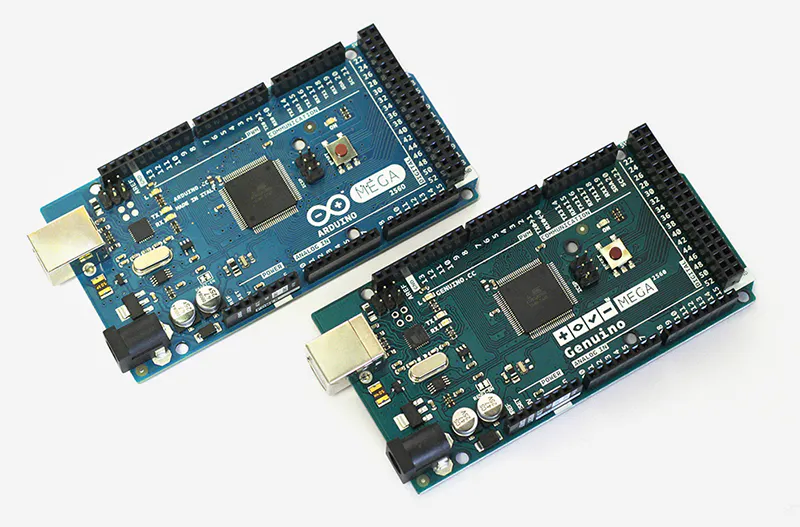Arduino Mega 2560 & Genuino Mega 2560 The Arduino Mega was used on the AiRobot. We needed the vast amount of pins!
×1Raspberry Pi 3 Model B We used the Raspberry Pi on the AiRobot to pull down information from the cloud and talk to the Arduino
×1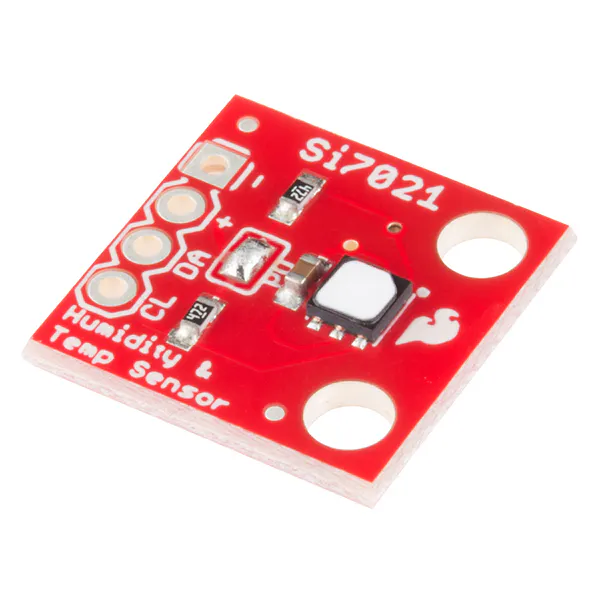SparkFun Humidity and Temperature Sensor Breakout - Si7021 The SparkFun Humidity and Temperature sensors were used on the AiRobot and both AiRobot wall mount sensors!
×3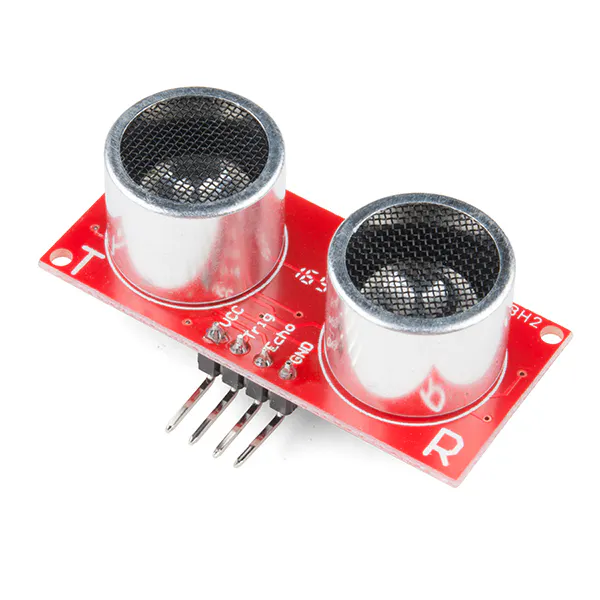SparkFun Ultrasonic Sensor - HC-SR04 3 Ultrasonic Sensors were used on the front of the AiRobot
×3
 PMS5003 - Air Quality Sensor The PMS5003 Air Quality Sensor gives us lots of great data on particle concentration and size distribution! We chose it over the Shinyei Air Quality Sensor for those reasons.
×3
 L298N Motor Drive Controller Board 2 motor drive controllers were used to control all 4 of our motors.
×2
 12V 10.5Ah Car Battery 12V 10.5Ah Lead Acid Battery was a great power system for our robot. We built the system to run for about an hour based on theoretical power consumption calculations!
×1
 Inverter This is an inverter that we used to step up 12V DC to 110V AC. This allows us to power any appliances we put on top of AiRobot.
×1
 Air Purifier We used this air purifier on the AiRobot!
×1
 Humidifier We used this humidifier for the AiRobot testing!
×1
 Lasko Ceramic Tower Heater We used this Ceramic Tower Heater for the AiRobot testing!
×1
 Pre-Cut Wooden Plaque We used these wooden plaques for the AiRobot chassis and the platform for the appliances to sit on.
×2
 Pololu 131:1 Metal Gearmotor We used 4 high torque 12V motors from Pololu. We wanted more torque because the robot might take heavy loads and does not need to move very quickly!
×4
 Pololu Stamped Aluminum L-Bracket L-Bracket used to secure motors onto wooden frame
×4
 Pololu Scooter/Skate Wheel 70×25mm These are the wheels we chose for our robot. We chose them mainly because we wanted to support heavier loads and we knew that we would be testing on hardwood floors. if you're interested in building this robot, and want it to perform better on different terrains, change the wheel size and type.
×4
 Pololu Aluminum Scooter Wheel Adapter This is used to attach the wheel to the motor!
×4
 M3 Standoffs We used M3 Standoffs to separate the chassis from the appliance platform on the AiRobot!
×1
 Zip Ties Zip Ties were used to secure objects and wires onto the frame.
×1Jumper wires (generic) **We used Male to Male, Male to Female, and Female to Female jumper wires!**
×1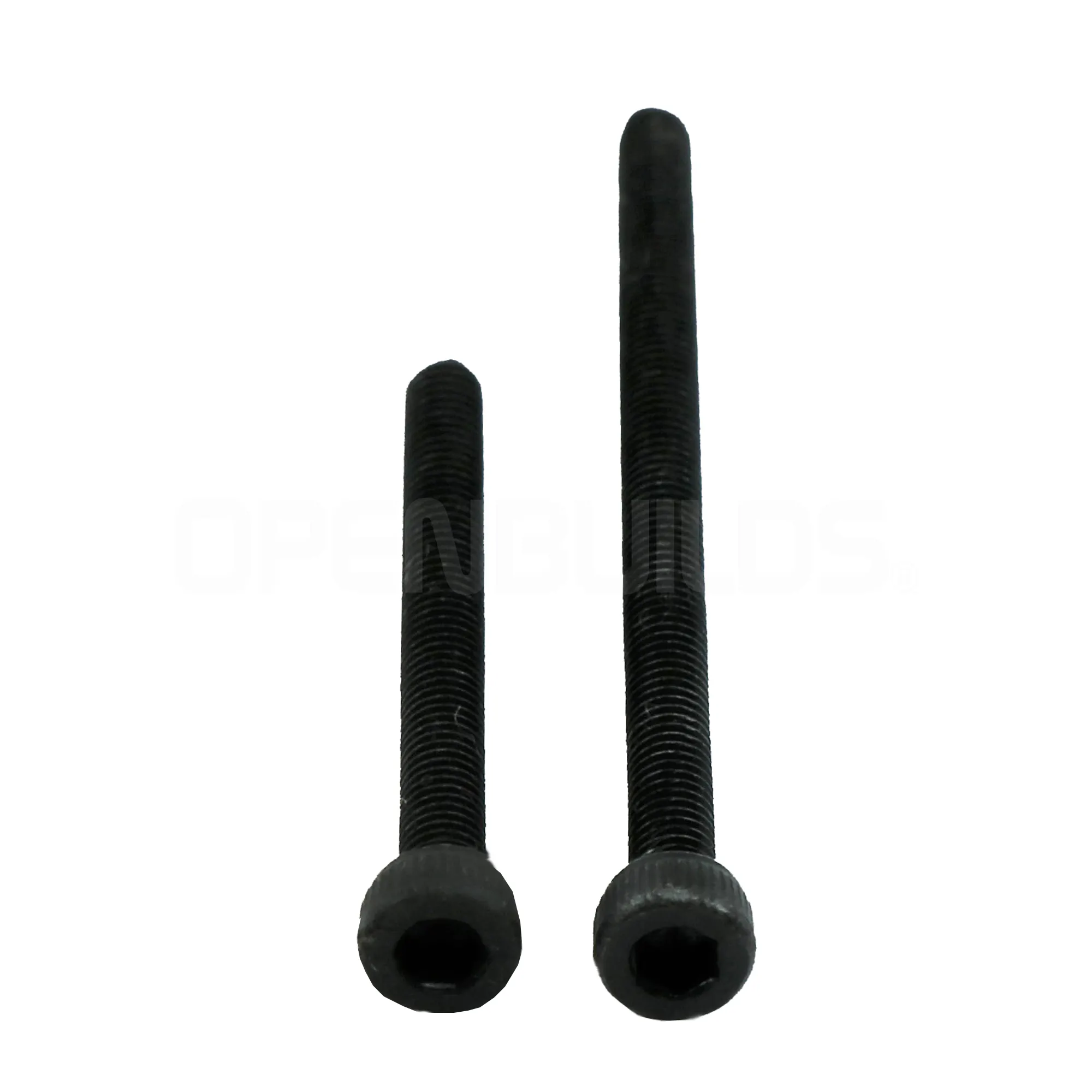OpenBuilds M3 Cap Head Screws Lots and Lots of M3 Screws!
×1OSH Park Custom fabricated PCB We used a custom PCB so we did not have to worry about wires going everywhere! Note that when you buy 1 batch from OSH Park, you get 3 PCB's! The Custom Made PCB Information is available in our project.
×2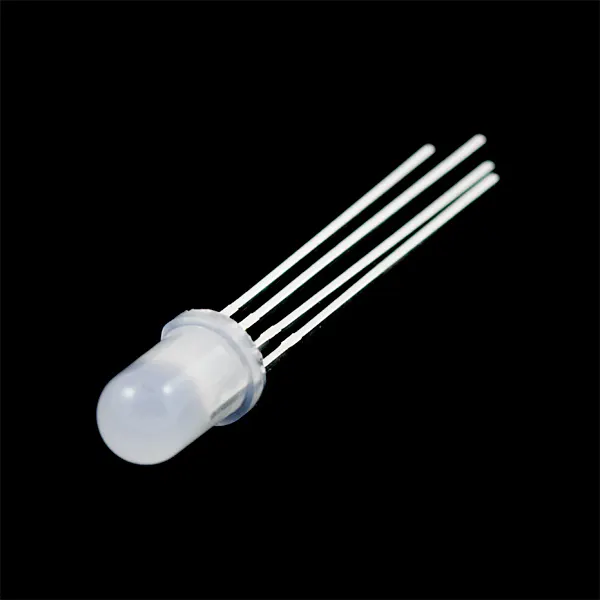RGB Diffused Common Cathode RGB LED is used on the custom PCB for indications
×2
 Green LED Green LED is used to indicate if the board is on or not
×2
 L7805 - 5V Regulator This was used to step down the 9V power for the AiRobot wall mount sensors
×2
 270 Ohm Resistor Resistors used on the custom PCB for the RGB LED and Power Indicator LED
×8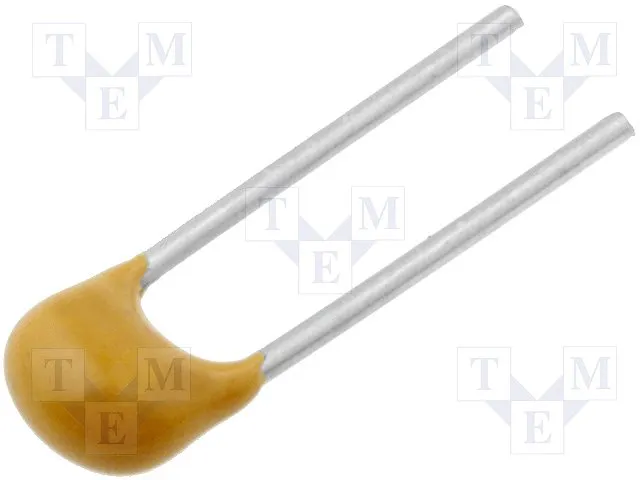Capacitor 100 nF Used to clean the 5V output signal for the L7805 on the AiRobot wall mount sensors
×2
 0.33 uf Capacitor Used to clean the 9V input for the L7805 on the AiRobot wall mount sensors
×2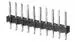Male Header 40 Position 1 Row (0.1") LOTS of header pins for the 2 Arduino Uno's on the AiRobot wall mount sensor pcb's!
×5
 Barrel Jack Connector (Female, PCB) Barrel Jack connector is used to connect the Battery Packs to the AiRobot wall mount sensors
×2
 9v Battery Holder The 9V Battery Holder is used to power the AiRobot wall mount sensor PCBs
×2
 12V 30A SPDT Switch or better
×1
 Barrel Jack (Female) w/ red+black stripped wires
×1
 Terminal Block to Barrel Jack (Male)
×1

### Software apps and online services

 Raspbian
 Python
 PySerial - Python communication library
 matplotlib (Python)
 numpy (Python)Arduino IDEHelium Dashboard
 DipTrace - PCB editing software

### Hand tools and fabrication machines

 Power DrillSoldering iron (generic)3D Printer (generic) 3D Printer is used to print the mounts and cases for: 2x Wallmount Sensor,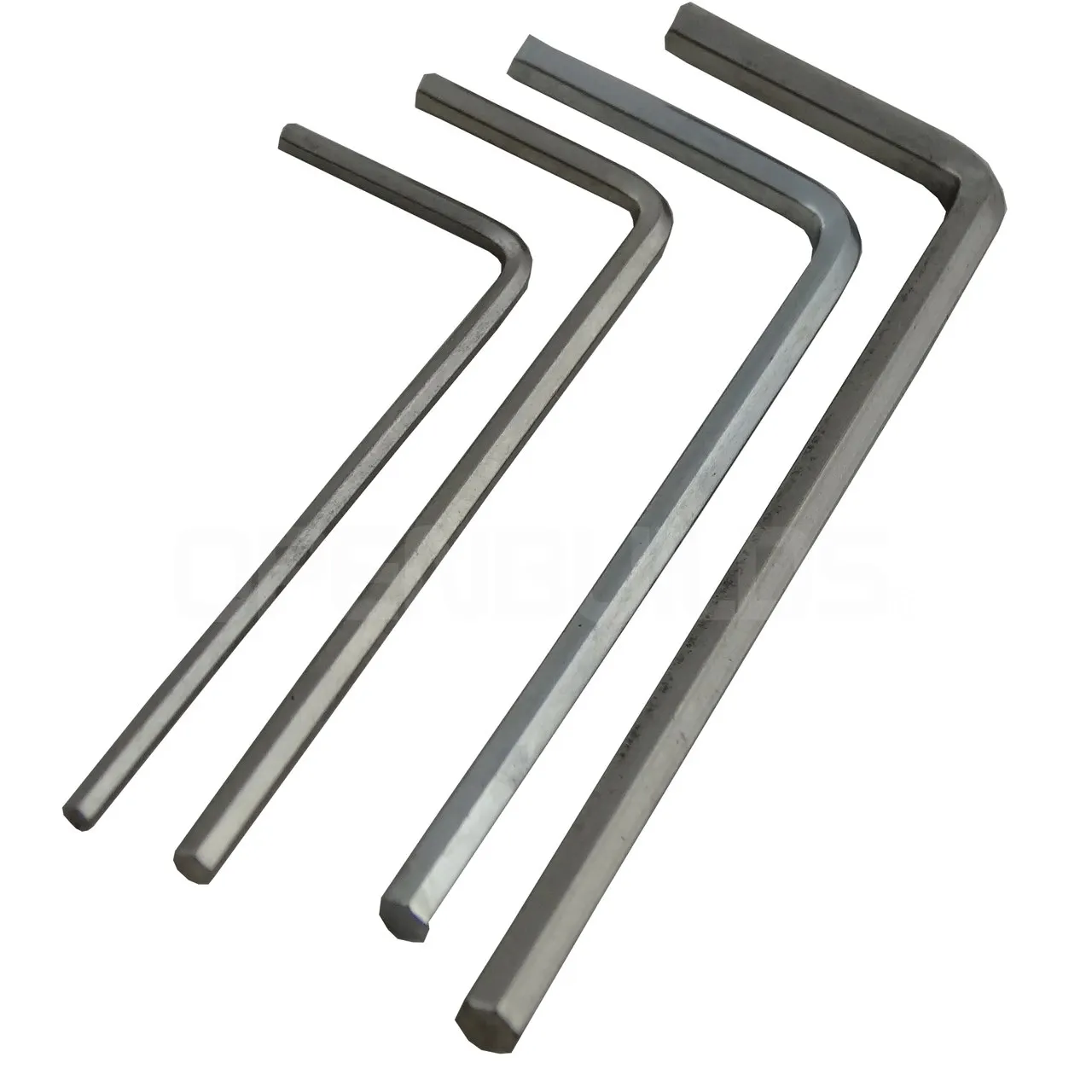OpenBuilds Allen Wrench
 Wire Stripper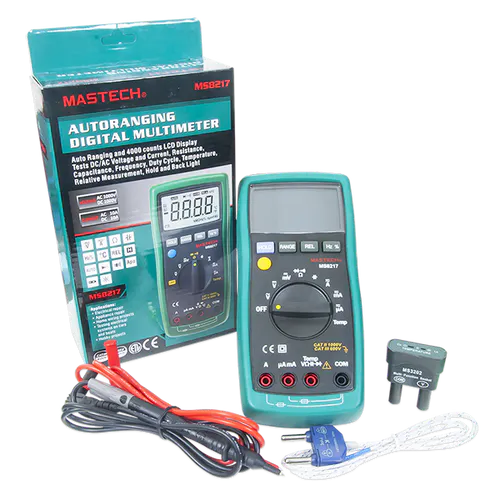Digilent Mastech MS8217 Autorange Digital Multimeter

## Custom parts and enclosures

### Si7021 Mount

This is used to mount the Si7021 Temperature+Humidity sensor on the AiRobot. That material used was ABS!
*****Note: it does not fit perfectly because we did not have the sensor when it was designed, nor the time to go back and fix it.

### Ultrasonic Mount

This is used to mount the Ultrasonic Sensors to the AiRobot. Material used was ABS!

### AiRobot Wallmount Sensor PCB

This is a DipTrace board file for the AiRobot Wallmount Sensor PCB. This allowed us to avoid messy wires!

## Schematics

### AiRobot Wallmount Sensor PCB Schematic (DipTrace)

This schematic file was created using DipTrace!

## Code

### AiRobot_final_code.ino

Arduino
Code ran on arduino mega. This is the brain of the robot and a LARGE code. Reads serial from raspberry and controls movements as well as uploading sensor data.
```//Libraries
#include "SparkFun_Si7021_Breakout_Library.h"
#include <Wire.h>
#include <Helium.h>
#include <SoftwareSerial.h>
#include "Arduino.h"
#include "Board.h"
#include "Helium.h"
#include "HeliumUtil.h"

//Definitions - Encoder A and B

//Code for pin names
//First digit: R,L for right and left
//Second digit: F,B for front and back
//Third digit: A,B for encoder A and encoder B
#define RFA 18
#define RFB 19
#define RBA 50
#define RBB 51
#define LFA 2 //LFA PIN CHANGED FROM 42 to 2
#define LFB 3 //LFB PIN CHANGED FROM 43 TO 3
#define LBA 48
#define LBB 49

//Definitions - Enable Pins
#define RFE 11 //RFE PIN CHANGED from 2 TO 11
#define RBE 9 //RBE PIN CHANGED FROM 3 to 9
#define LFE 4
#define LBE 5

//Definitions - Motor Poles
#define RF1 26
#define RF2 27
#define RB1 28
#define RB2 29
#define LF1 7
#define LF2 8
#define LB1 32
#define LB2 33

//Definitions - Encoders
int RFval;
int RBval;
int LFval;
int LBval;
int RFpos = 0;
int RBpos = 0;
int LFpos = 0;
int LBpos = 0;
int RFlast = LOW;
int RBlast = LOW;
int LFlast = LOW;
int LBlast = LOW;
int RFn = LOW;
int RBn = LOW;
int LFn = LOW;
int LBn = LOW;

// wheel information definitions
const int diameter = 70; // in mm
const int pi = 3.141592654;
const int circumference = diameter * pi;
const int tickspercm = 360 / (diameter*pi);

//---------------------------------------

//Setup Serial Communication int
String Direction;           //the direction that the roobt will drive in
String distance;               //the distance you want to travel
String angle;

//Ultrasonic Sensors
const int trigPin1 = 36;
const int echoPin1 = 37;
const int trigPin2 = 38;
const int echoPin2 = 39;
const int trigPin3 = 40;
const int echoPin3 = 41;
// defines variables
long duration1;
int distance1;
long duration2;
int distance2;
long duration3;
int distance3;
int Boundary = 20;

bool first_on = true;
int RF_pos_copy = 0;
int TURNS = 0;

//Setup Software Serial for Sensors
SoftwareSerial pmsSerial(10, 11);
Weather sensor;
//Set initial Float Values
float humidity = 0;
float tempf = 0;

//Helium Channel
Helium  helium(&atom_serial);
Channel channel(&helium);

void report_status(int status)
{
if (helium_status_OK == status)
{
Serial.println("Succeeded");
}
else
{
Serial.println("Failed");
}
}

void report_status_result(int status, int result)
{
if (helium_status_OK == status)
{
if (result == 0)
{
Serial.println("Succeeded");
}
else {
Serial.print("Failed - ");
Serial.println(result);
}
}
else
{
Serial.println("Failed");
}
}

void setup() {
Serial.begin(9600);
//HELIUM Initialization
// Begin communication with the Helium Atom
// The baud rate differs per supported board
// and is configured in Board.h
helium.begin(HELIUM_BAUD_RATE);

// Connect the Atom to the Helium Network
Serial.print("Connecting - ");
int status = helium.connect();
int8_t result;
Serial.print("Creating Channel - ");
status = channel.begin("env-hub", &result);

// Print status and result
report_status_result(status, result);

//PMS5003 Baud Rate is 9600
pmsSerial.begin(9600);
//Initialize the Si7021 and ping it
sensor.begin();

//Set Outputs
pinMode(RFA, INPUT); pinMode(RFB, INPUT);
pinMode(RBA, INPUT); pinMode(RBB, INPUT);
pinMode(LFA, INPUT); pinMode(LFB, INPUT);
pinMode(LBA, INPUT); pinMode(LBB, INPUT);

pinMode(RFE, OUTPUT); pinMode(RBE, OUTPUT);
pinMode(LFE, OUTPUT); pinMode(LBE, OUTPUT);

pinMode(RF1, OUTPUT); pinMode(RF2, OUTPUT);
pinMode(RB1, OUTPUT); pinMode(RB2, OUTPUT);
pinMode(LF1, OUTPUT); pinMode(LF2, OUTPUT);
pinMode(LB1, OUTPUT); pinMode(LB2, OUTPUT);

pinMode(trigPin1, OUTPUT); // Sets the trigPin as an Output
pinMode(echoPin1, INPUT); // Sets the echoPin as an Input
pinMode(trigPin2, OUTPUT); // Sets the trigPin as an Output
pinMode(echoPin2, INPUT); // Sets the echoPin as an Input
pinMode(trigPin3, OUTPUT); // Sets the trigPin as an Output
pinMode(echoPin3, INPUT); // Sets the echoPin as an Input

//Motor Related Initilizations
digitalWrite(RFA, LOW); digitalWrite(RFB, LOW);
digitalWrite(RBA, LOW); digitalWrite(RBB, LOW);
digitalWrite(LFA, LOW); digitalWrite(LFB, LOW);
digitalWrite(LBA, LOW); digitalWrite(LBB, LOW);

digitalWrite(RFE, LOW); digitalWrite(RBE, LOW);
digitalWrite(LFE, LOW); digitalWrite(LBE, LOW);

digitalWrite(RF1, LOW); digitalWrite(RF2, LOW);
digitalWrite(RB1, LOW); digitalWrite(RB2, LOW);
digitalWrite(LF1, LOW); digitalWrite(LF2, LOW);
digitalWrite(LB1, LOW); digitalWrite(LB2, LOW);

//Initialize the Si7021
//sensor.begin(); //WE CHANGED THIS AND WE NEED TO GET IT RUNNING

}

struct pms5003data {
uint16_t framelen;
uint16_t pm10_standard, pm25_standard, pm100_standard;
uint16_t pm10_env, pm25_env, pm100_env;
uint16_t particles_03um, particles_05um, particles_10um, particles_25um, particles_50um, particles_100um;
uint16_t unused;
uint16_t checksum;
};

struct pms5003data data;

void loop() {
if (Serial.available() > 0)                         //if the user has sent a command
{
Direction = Serial.readStringUntil(' ');       //read the characters in the command until you reach the first space
distance = Serial.readStringUntil(' ');           //read the characters in the command until you reach the second space
//print the command that was just received in the serial monitor
Serial.print(Direction);
Serial.print(" ");
Serial.println(distance.toInt());

if (Direction == "m")                         //if the entered direction is forward
{
Move(distance.toInt());
}
if (Direction == "t")                         //if the entered direction is forward
{
Turn(distance.toInt());
}
if (Direction == "r")                         //if the entered direction is forward
{
if (distance.toInt()==0){
//monitor serial for movement commands
//once at desired location then run r 1,2,3 depending on desired sensor
Serial.println("waiting for commands")
while (true) {
if (Serial.available() > 0)                         //if the user has sent a command
{
Direction = Serial.readStringUntil(' ');       //read the characters in the command until you reach the first space
distance = Serial.readStringUntil(' ');           //read the characters in the command until you reach the second space
//print the command that was just received in the serial monitor
Serial.print(Direction);
Serial.print(" ");
Serial.println(distance.toInt());

if (Direction == "m")                         //if the entered direction is forward
{
Move(distance.toInt());
}
if (Direction == "t")                         //if the entered direction is forward
{
Turn(distance.toInt());
}
if (Direction == "r")                         //if the entered direction is forward
{
if (distance.toInt()>0){

if (distance.toInt()==1){
char buffer;
while (true){
tempf = sensor.getTempF();
String T = dtostrf(tempf, 0, 2, buffer);
int8_t result;

String HeliumData="1111,"+ T;
const char * Data = HeliumData.c_str();
Serial.println(Data); //Test Diagnostic
int status = channel.send(Data, strlen(Data), &result);
}
}

if (distance.toInt()==2){
char buffer;
while (true){
humidity = sensor.getRH();
String H = dtostrf(humidity, 0, 2, buffer);
int8_t result;

String HeliumData="1111,"+ H;
const char * Data = HeliumData.c_str();
Serial.println(Data); //Test Diagnostic
int status = channel.send(Data, strlen(Data), &result);
}
}

if (distance.toInt()==3){
char buffer;
while (true){

/*
// Size ranges of particles - Good for histogram plot
// Particles > 0.3um / 0.1L air
String PM_03um = dtostrf(data.particles_03um, 0, 0, buffer);
//Particles > 0.5um / 0.1L air
String PM_05um = dtostrf(data.particles_05um, 0, 0, buffer);
//Particles > 1.0um / 0.1L air
String PM_10um = dtostrf(data.particles_10um, 0, 0, buffer);
//Particles > 2.5um / 0.1L air
String PM_25um = dtostrf(data.particles_25um, 0, 0, buffer);
//Particles > 5.0um / 0.1L air
String PM_50um = dtostrf(data.particles_50um, 0, 0, buffer);
//Particles > 50 um / 0.1L air
String PM_100um = dtostrf(data.particles_100um, 0, 0, buffer);
*/

// Concentration Units (standard)
// PM 1.0
//String PM1 = dtostrf(data.pm10_standard, 0, 0, buffer);
// PM 2.5
String PM2p5 = dtostrf(data.pm25_standard, 0, 0, buffer);
// PM 10
//String PM10 = dtostrf(data.pm100_standard, 0, 0, buffer);

int8_t result;

String HeliumData="1111,"+ PM2p5;
const char * Data = HeliumData.c_str();
Serial.println(Data); //Test Diagnostic
int status = channel.send(Data, strlen(Data), &result);
}
}
}

}
}
}
}
}
}
}
else
{
//blah                               //turn the motors
}
humidity = sensor.getRH();
tempf = sensor.getTempF();

//----------------------------------------------------------
// String Section
String SensorName = "1111"; //2222,3333,etc.
char buffer;
// Size ranges of particles - Good for histogram plot
// Particles > 0.3um / 0.1L air
String PM_03um = dtostrf(data.particles_03um, 0, 0, buffer);
//Particles > 0.5um / 0.1L air
String PM_05um = dtostrf(data.particles_05um, 0, 0, buffer);
//Particles > 1.0um / 0.1L air
String PM_10um = dtostrf(data.particles_10um, 0, 0, buffer);
//Particles > 2.5um / 0.1L air
String PM_25um = dtostrf(data.particles_25um, 0, 0, buffer);
//Particles > 5.0um / 0.1L air
String PM_50um = dtostrf(data.particles_50um, 0, 0, buffer);
//Particles > 50 um / 0.1L air
String PM_100um = dtostrf(data.particles_100um, 0, 0, buffer);

// Concentration Units (standard)
// PM 1.0
String PM1 = dtostrf(data.pm10_standard, 0, 0, buffer);
// PM 2.5
String PM2p5 = dtostrf(data.pm25_standard, 0, 0, buffer);
// PM 10
String PM10 = dtostrf(data.pm100_standard, 0, 0, buffer);

//Temperature RH
String Tempf = dtostrf(tempf, 0, 2, buffer);
String Humidity = dtostrf(humidity, 0, 2, buffer);

//Convert all strings into One String to rule them all. **********USE THIS STRING TO SEND TO HELIUM!***********
int8_t result;
if (first_on==true){
first_on=false;
String HeliumData="1111,-9999,0,0,0,0,0,0,0,0,0,0,0";
const char * Data = HeliumData.c_str();
Serial.println(Data); //Test Diagnostic
delay(1);
int status = channel.send(Data, strlen(Data), &result);
}
String HeliumData = SensorName + "," + Tempf + "," + Humidity + PM_03um + "," + PM_05um + "," + PM_10um + "," + PM_25um + "," + PM_50um + "," + PM_100um + "," + PM1 + "," + PM2p5 + "," + PM10 + ","+String(RF_pos_copy)+","+String(TURNS);
const char * Data = HeliumData.c_str();
Serial.println(Data); //Test Diagnostic
Serial.println(RF_pos_copy);
Serial.println(TURNS);
//after turns gets uploaded set back to 0
TURNS=0;
// Send data to channel
//const char * SendData = HeliumData;
int status = channel.send(Data, strlen(Data), &result);
//report_status_result(status, result);
}
}

if (! s->available()) {
return false;
}

// Read a byte at a time until we get the'0x42' start-byte
if (s->peek() != 0x42) {
return false;
}

if (s->available() < 32) {
return false;
}

uint8_t buffer;
uint16_t sum = 0;

for (uint8_t i=0; i<30; i++) {
sum += buffer[i];
}
uint16_t buffer_u16;
for (uint8_t i=0; i<15; i++) {
buffer_u16[i] = buffer[2 + i*2 + 1];
buffer_u16[i] += (buffer[2 + i*2] << 8);
}
memcpy((void *)&data, (void *)buffer_u16, 30);

if (sum != data.checksum) {
//Serial.println("Checksum failure");
return false;
}
return true;
}
//
void Move(int distance)                       //function for driving the right motor
{
digitalWrite(RFE, HIGH); digitalWrite(RBE, HIGH);
digitalWrite(LFE, HIGH); digitalWrite(LBE, HIGH);
if (distance > 0) {
while (RFpos < distance) {
if ((RFlast == LOW) && (RFn == HIGH)) {
RFpos--;
RF_pos_copy--;
}
else {
RFpos++;
RF_pos_copy++;
}
if((distance2<Boundary and distance2>0) or (distance1<Boundary and distance1>0) or (distance3<Boundary and distance3>0)){ //or distance1<Boundary or distance3<Boundary
Serial.print(distance2);
Serial.println(",OBJECT!");
RFpos=0;
distance2=0;
Stop();
break;
}
Serial.println(RFpos);
Serial.println(RF_pos_copy);

//        Serial.print(",");
//        Serial.print(distance1);
//        Serial.print(",");
//        Serial.print(distance2);
//        Serial.print(",");
//        Serial.println(distance3);

}
RFlast = RFn;

digitalWrite(RF1, HIGH);
digitalWrite(RB2, HIGH);
digitalWrite(LF1, HIGH);
digitalWrite(LB2, HIGH);

digitalWrite(LF2, LOW);
digitalWrite(LB1, LOW);
digitalWrite(RF2, LOW);
digitalWrite(RB1, LOW);
}
RFpos = 0;
Stop();
}
if (distance < 0) {
//    analogWrite(RFE, 215); analogWrite(RBE, 215);
//    analogWrite(LFE, 215); analogWrite(LBE, 215);
while (RFpos > distance) {
if ((RFlast == LOW) && (RFn == HIGH)) {
RFpos--;
RF_pos_copy--;
}
else {
RFpos++;
RF_pos_copy++;
}
Serial.println(RFpos);
}
RFlast = RFn;

digitalWrite(RF1, LOW);
digitalWrite(RB2, LOW);
digitalWrite(LF1, LOW);
digitalWrite(LB2, LOW);

digitalWrite(LF2, HIGH);
digitalWrite(LB1, HIGH);
digitalWrite(RF2, HIGH);
digitalWrite(RB1, HIGH);
}
RFpos = 0;
Stop();
}
}
void Turn(int angle) {
digitalWrite(RFE, HIGH); digitalWrite(RBE, HIGH);
digitalWrite(LFE, HIGH); digitalWrite(LBE, HIGH);
if (angle > 0) {
while (RFpos < angle) {
if ((RFlast == LOW) && (RFn == HIGH)) {
RFpos--;
}
else {
RFpos++;
}

Serial.println(RFpos);
//Serial.print(",");
}

RFlast = RFn;

digitalWrite(RF2, LOW);
digitalWrite(RB1, LOW);
digitalWrite(LF1, LOW);
digitalWrite(LB2, LOW);

digitalWrite(RF1, HIGH);
digitalWrite(RB2, HIGH);
digitalWrite(LF2, HIGH);
digitalWrite(LB1, HIGH);
}
if (RFpos>90){
TURNS=1;}
if (RFpos<-90) {TURNS=-1;}
RFpos = 0;
Stop();
}
if (angle < 0) {
while (RFpos > angle) {
if ((RFlast == LOW) && (RFn == HIGH)) {
RFpos--;
}
else {
RFpos++;
}

Serial.println(RFpos);
}

RFlast = RFn;

digitalWrite(RF1, LOW);
digitalWrite(RB2, LOW);
digitalWrite(LF2, LOW);
digitalWrite(LB1, LOW);

digitalWrite(RF2, HIGH);
digitalWrite(RB1, HIGH);
digitalWrite(LF1, HIGH);
digitalWrite(LB2, HIGH);
}
if (RFpos>90){
TURNS=1;}
if  (RFpos<-90){TURNS=-1;}
RFpos = 0;
Stop();
}
}
void Stop() {
digitalWrite(LF2, LOW);
digitalWrite(LB1, LOW);
digitalWrite(RF2, LOW);
digitalWrite(RB1, LOW);
digitalWrite(RF1, LOW);
digitalWrite(RB2, LOW);
digitalWrite(LF1, LOW);
digitalWrite(LB2, LOW);
delay(250);

}
int Ultrasonic() {
// Clears the trigPin
digitalWrite(trigPin1, LOW);
delayMicroseconds(2);
// Sets the trigPin on HIGH state for 10 micro seconds
digitalWrite(trigPin1, HIGH);
delayMicroseconds(10);
digitalWrite(trigPin1, LOW);
// Reads the echoPin, returns the sound wave travel time in microseconds
duration1 = pulseIn(echoPin1, HIGH);
// Calculating the distance
distance1 = duration1 * 0.034 / 2;
// Prints the distance on the Serial Monitor
//Serial.print("Distance1: ");
//Serial.print(distance1);
//Serial.print(",");
delay(10);

// Clears the trigPin
digitalWrite(trigPin2, LOW);
delayMicroseconds(2);
// Sets the trigPin on HIGH state for 10 micro seconds
digitalWrite(trigPin2, HIGH);
delayMicroseconds(10);
digitalWrite(trigPin2, LOW);
// Reads the echoPin, returns the sound wave travel time in microseconds
duration2 = pulseIn(echoPin2, HIGH);
// Calculating the distance
distance2 = duration2 * 0.034 / 2;
// Prints the distance on the Serial Monitor
//Serial.print("Distance2: ");
//Serial.print(distance2);
//Serial.print(",");
delay(10);

// Clears the trigPin
digitalWrite(trigPin3, LOW);
delayMicroseconds(2);
// Sets the trigPin on HIGH state for 10 micro seconds
digitalWrite(trigPin3, HIGH);
delayMicroseconds(10);
digitalWrite(trigPin3, LOW);
// Reads the echoPin, returns the sound wave travel time in microseconds
duration3 = pulseIn(echoPin3, HIGH);
// Calculating the distance
distance3 = duration3 * 0.034 / 2;
// Prints the distance on the Serial Monitor
//Serial.print("Distance3: ");
//Serial.println(distance3);
delay(10);

}
```

### board.h

Arduino
File required to upload arduino mega code.
```/*
* Copyright 2017, Helium Systems, Inc.
*/

#ifndef BOARD_H
#define BOARD_H

#if defined(ARDUINO_AVR_UNO)
#include "SoftwareSerial.h"
SoftwareSerial atom_serial(8, 9);
#define HELIUM_BAUD_RATE helium_baud_b9600

#elif defined(ARDUINO_AVR_MEGA2560)
#include "SoftwareSerial.h"
SoftwareSerial atom_serial(10,11);
#define HELIUM_BAUD_RATE helium_baud_b9600

#elif defined(ARDUINO_SAM_ZERO)
// Arduino M0 Pro
#define atom_serial Serial5

#elif defined(ARDUINO_SAMD_ZERO)
// Arduino Zero
#define atom_serial Serial1

#elif defined(ARDUINO_SAM_DUE)
// Arduino Due with Serial3 (pin 15, 14)
// mapped to pin 8, 9 on the adapter
#define atom_serial Serial3
#endif

#if defined(CORE_TEENSY)
//Teensy with Serial1 (pin 0, 1)
#define atom_serial Serial1
extern "C"{

int _write(int f, char *ptr,int len){
int i;
for(i=0;i<len;i++)
{
atom_serial.write(*ptr++);
}
return len;
}
int _read (int f, char *ptr, int len)
{

return len;
}
}

#elif defined(ARDUINO_AVR_PRO)
//ProMini/Micro with Serial pins (8,9)
#include "SoftwareSerial.h"
SoftwareSerial atom_serial(8,9);

#endif

#ifndef HELIUM_BAUD_RATE
#define HELIUM_BAUD_RATE helium_baud_b115200
#endif

#endif // BOARD_H
```

### data_monitor.py

Python
Code ran on raspberry pi to plot data changing over time after AiRobot has reached final destination.
```import os
import numpy as np
import matplotlib.pyplot as plt

os.system("gsutil cp gs://mybuckettest12345/data_monitor.txt /home/pi/Desktop/airobot/")
f=open("data_monitor.txt","r")
data=line[0:-1].split(',')
x=np.arange(0,len(data))
y=np.zeros((1,len(data)))
for i in range(0,len(data)):
y[i]=int(data[i])

print(x)
print(y)
plt.plot(y)
plt.show()
```

### manual_input_serial.py

Python
Code ran on Rpi to manually input serial information to control movements. Used primarily for debugging.
```import os
import serial
import time

ser=serial.Serial(port='/dev/ttyACM0',baudrate=9600)
time.sleep(5)

while True:
command=raw_input("Enter command: ")
ser.flushInput()
ser.write(command)
```

### walk_to_pollution.py

Python
Code ran on RPi that tells AiRobot where its final destination is so it can solve detected problem.
```import os
import serial
import time
import numpy as np

os.system("gsutil cp gs://mybuckettest12345/commands.txt /home/pi/Desktop/airobot/")
f=open("commands.txt","r")
commands=line[0:-1].split(',')
print(commands)

sleeptime=5
ser=serial.Serial(port='/dev/ttyACM0',baudrate=9600,parity=serial.PARITY_NONE,stopbits=serial.STOPBITS_ONE,bytesize=serial.EIGHTBITS,timeout=2)
time.sleep(sleeptime)
turn = '-325'
foot = '122'

print("Starting")
ser.flushInput()
ser.write('r 0')
time.sleep(sleeptime)

fc=0
for i in range(0,len(commands)):
ser.flushInput() #flush or youre fucked
if commands[i]=='t1':
com='t '+turn
print('turn')
if commands[i]=='m1':
com='m '+foot
print('forward')
fc+=1

ser.write(com)
time.sleep(sleeptime)
if fc==3:
ser.flushInput()
ser.write('t -15')
time.sleep(sleeptime)
fc=0

ser.flushInput()
ser.write('r 3')
print("At particle heavy location!")
print("Absorbing ...")
```

### rectangle_walk.py

Python
Code ran on virtual machine that tells AiRobot to walk in a rectangle to collect sensor data from around a room. This data is streamed to Google Cloud and used to create a heatmap.
```import os
import serial
import time
#make sure eto flush serial before writing to it to avoid an issues
#allow time for commands to be sent and processed

sleeptime=7
ser=serial.Serial(port='/dev/ttyACM0',baudrate=9600,parity=serial.PARITY_NONE,stopbits=serial.STOPBITS_ONE,bytesize=serial.EIGHTBITS,timeout=2)
time.sleep(sleeptime)
turn = '-325'
foot = '120'

print("Starting")
for i in range(0,4):
ser.flushInput()
ser.write('m '+foot)
time.sleep(sleeptime)

ser.flushInput()
ser.write('t '+turn)
time.sleep(sleeptime)

for i in range(0,4):
ser.flushInput()
ser.write('m '+foot)
time.sleep(sleeptime)

ser.flushInput()
time.sleep(sleeptime)

for i in range(0,3):
ser.flushInput() #flush or youre fucked
ser.write('m '+foot)
time.sleep(sleeptime)

ser.flushInput() #flush or youre fucked
ser.write('t '+turn)
time.sleep(sleeptime)

for i in range(0,4):
ser.flushInput() #flush or youre fucked
ser.write('m '+foot)
time.sleep(sleeptime)

ser.flushInput()
ser.write('t '+turn)
time.sleep(sleeptime)

for i in range(0,4):
ser.flushInput()
ser.write('m '+foot)
time.sleep(sleeptime)

ser.flushInput()
time.sleep(sleeptime)

for i in range(0,3):
ser.flushInput()
ser.write('m '+foot)
time.sleep(sleeptime)

ser.close()
print("done")

```

### cronthon.py

Python
Code ran on virtual machine inside cron job which allows for processing of data and heatmap creation.
```import  time
import os

# Function to gran current time
def get_time():
return time.strftime("%Y-%m-%d %H:%M:%S", time.gmtime())

# Sleep for 14 seconds to allow for GCS to create data files
#time.sleep(14)
current_time=str(get_time())
# For debugging
print(current_time)
print(current_time[-5:-3])
print(int(current_time[-5:-3])%5)
if int(current_time[-5:-3])%5==0:
with open(filename, "w") as f:
# Write data to file.
f.write(current_time)
f.write("\n")
f.write("Great Success")
time.sleep(5)
```

### heatmap_generator.py

Python
Code ran on virtual machine that creates heatmaps and generates a file called commands that tells AiRobot where to go to reduce pollution. Currently set on air quality.
```import numpy as np
import time
import os
import glob
import matplotlib
matplotlib.use('Agg')
import matplotlib.pyplot as plt
import matplotlib.path as mpath
import random
import copy as cp

# Remove all old files from VM instance
for f in del_files:
os.remove(f)

# Copy in new files from GCS

# Remove all GCS txt files
#os.system("gsutil rm gs://mybuckettest12345/data/*")

# Read each line of the new files
lines=[]
for filename in os.listdir(os.getcwd()):
new_lines=[line.rstrip('\n') for line in open(filename)]
for j in range(0,len(new_lines)):
lines.append(new_lines[j].split(','))

all_data=np.zeros((len(lines),13))
for i in range(0,len(lines)):
all_data[i]=float(lines[i])
all_data[i]=float(lines[i])
all_data[i]=float(lines[i])
all_data[i]=float(lines[i])
all_data[i]=float(lines[i])
all_data[i]=float(lines[i])
all_data[i]=float(lines[i])
all_data[i]=float(lines[i])
all_data[i]=float(lines[i])
all_data[i]=float(lines[i])
all_data[i]=float(lines[i])
all_data[i]=float(lines[i])
all_data[i]=float(lines[i])
# Filter data into S1,S2,S3: Array for each sensor
S1=all_data[all_data[:,0] == 1111]
S2=all_data[all_data[:,0] == 2222]
S3=all_data[all_data[:,0] == 3333]

# delete all data before row in S1 with only len=2
append=False
create=True
clean_S1=np.zeros((len(S1),13))
ff=0
for i in range(ff,len(S1)-2):
if append==True:
if create==True:
strt=i
clean_S1=np.zeros((len(S1)-strt-2,13))
create=False
clean_S1[i-strt]=S1[i]
clean_S1[i-strt]=S1[i]
clean_S1[i-strt]=S1[i]
clean_S1[i-strt]=S1[i]
clean_S1[i-strt]=S1[i]
clean_S1[i-strt]=S1[i]
clean_S1[i-strt]=S1[i]
clean_S1[i-strt]=S1[i]
clean_S1[i-strt]=S1[i]
clean_S1[i-strt]=S1[i]
clean_S1[i-strt]=S1[i]
clean_S1[i-strt]=S1[i]
clean_S1[i-strt]=S1[i]

if S1[i+2]!=0 and append==False:
append=True

S1_pos=np.zeros((len(clean_S1),2))
# need to clean up encoder and turn information and convert to position
tpf=120
direction=[1,0]
pos=[0,0]
for i in range(1,len(clean_S1)):
turn=clean_S1[i]
#update position
pos=pos+direction*(clean_S1[i]-clean_S1[i-1])/tpf
pos=pos+direction*(clean_S1[i]-clean_S1[i-1])/tpf

S1_pos[i]=pos
S1_pos[i]=pos
#update direction
if turn==1: #CCW
if direction==[1,0]:
direction=[0,1]
elif direction==[-1,0]:
direction=[0,-1]
elif direction==[0,1]:
direction=[-1,0]
elif direction==[0,-1]:
direction=[1,0]
if turn==-1: #CW
if direction==[1,0]:
direction=[0,-1]
elif direction==[-1,0]:
direction=[0,1]
elif direction==[0,1]:
direction=[1,0]
elif direction==[0,-1]:
direction=[-1,0]

print(S1_pos)

# y,x
S2_loc=[3,5]
S3_loc=[-9,-1]
#Create meshgrid
mesh_square=1 # in ft
# these values need to be rounded to nearest half foot (or foot depends on resolution)
S1xmin=int(round(np.amin(S1_pos[:,0])))
S1xmax=int(round(np.amax(S1_pos[:,0])))
S1ymin=int(round(np.amin(S1_pos[:,1])))
S1ymax=int(round(np.amax(S1_pos[:,1])))
#determine if sensors are mins or maxs compared to robot
xmin=min(S1xmin,S2_loc,S3_loc)
xmax=max(S1xmax,S2_loc,S3_loc)
ymin=min(S1ymin,S2_loc,S3_loc)
ymax=max(S1ymax,S2_loc,S3_loc)

x=np.arange(int(xmin/mesh_square)-mesh_square,int(xmax/mesh_square)+mesh_square,mesh_square)
y=np.arange(int(ymin/mesh_square)-mesh_square,int(ymax/mesh_square)+mesh_square,mesh_square)
xx,yy=np.meshgrid(x,y)
w,h=xx.shape
# NOTE: heatmap plot cuts off last row and column so ignore it
Z_temp=np.zeros((w, h))
Z_humid=np.zeros((w, h))
Z_particle=np.zeros((w, h))
for i in range(0,len(clean_S1)):
Z_temp[int(S1_pos[i]/mesh_square)-ymin][int(S1_pos[i]/mesh_square)-xmin]=clean_S1[i]
Z_humid[int(S1_pos[i]/mesh_square)-ymin][int(S1_pos[i]/mesh_square)-xmin]=clean_S1[i]
Z_particle[int(S1_pos[i]/mesh_square)-ymin][int(S1_pos[i]/mesh_square)-xmin]=clean_S1[i]

Z_temp[int(S2_loc/mesh_square)-ymin][int(S2_loc/mesh_square)-xmin]=S2
Z_temp[int(S3_loc/mesh_square)-ymin][int(S3_loc/mesh_square)-xmin]=S3
Z_humid[int(S2_loc/mesh_square)-ymin][int(S2_loc/mesh_square)-xmin]=S2
Z_humid[int(S3_loc/mesh_square)-ymin][int(S3_loc/mesh_square)-xmin]=S3
Z_particle[int(S2_loc/mesh_square)-ymin][int(S2_loc/mesh_square)-xmin]=S2
Z_particle[int(S3_loc/mesh_square)-ymin][int(S3_loc/mesh_square)-xmin]=S3
# Filling in all the values

# algorithm goes through all "empty" points and sets equal to average of 8 neighbors or closest value
loop=True
while loop==True:
loop=False
Z_copy_temp=cp.deepcopy(Z_temp)
Z_copy_humid=cp.deepcopy(Z_humid)
Z_copy_particle=cp.deepcopy(Z_particle)
for i in range(0,w-1):
for j in range(0,h-1):
if Z_copy_temp[i][j]==0:
#find average of 8 neighbors
avg_temp=0
avg_humid=0
avg_particle=0
avg_cnt=0
rng=1
for row in range(-rng,rng+1):
for col in range(-rng,rng+1):
try:
val=Z_copy_temp[i+row][j+col]
if val!=0:
avg_temp+=Z_copy_temp[i+row][j+col]
avg_humid+=Z_copy_humid[i+row][j+col]
avg_particle+=Z_copy_particle[i+row][j+col]
avg_cnt+=1
except:
pass

if avg_cnt==0:
loop=True
else:
Z_temp[i][j]=avg_temp/avg_cnt
Z_humid[i][j]=avg_humid/avg_cnt
Z_particle[i][j]=avg_particle/avg_cnt

fig_temp=plt.figure()
pc_temp=ax_temp.pcolormesh(xx,yy,Z_temp,cmap='hot')
fig_temp.colorbar(pc_temp)
ax_temp.set_title('Temperature Heatmap')
plt.show()

timestr = time.strftime("%Y_%m_%d_%H_%M_%S")
heatmap_name_temp='heatmap_temp_'+timestr
plt.savefig(heatmap_name_temp)

fig_humid=plt.figure()
pc_humid=ax_humid.pcolormesh(xx,yy,Z_humid,cmap='hot')
fig_humid.colorbar(pc_humid)
ax_humid.set_title('Humidity Heatmap')
plt.show()
heatmap_name_humid='heatmap_humid_'+timestr
plt.savefig(heatmap_name_humid)

fig_particle=plt.figure()
pc_particle=ax_particle.pcolormesh(xx,yy,Z_particle,cmap='hot')
fig_particle.colorbar(pc_particle)
ax_particle.set_title('Particle Heatmap')
plt.show()
heatmap_name_particle='heatmap_particle_'+timestr
plt.savefig(heatmap_name_particle)

os.system("gsutil cp "+heatmap_name_temp+".png gs://mybuckettest12345/")
os.system("gsutil cp "+heatmap_name_humid+".png gs://mybuckettest12345/")
os.system("gsutil cp "+heatmap_name_particle+".png gs://mybuckettest12345/")

# time to locate and travel to problem area
# using particle sensor

max_val=np.amax(Z_particle)
for i in range(0,int(xmax-xmin+1)):
for j in range(0,int(ymax-ymin+1)):
if Z_particle[j][i]==max_val:
particle_loc=[i+xmin,j+ymin]
print(i,j)
print(xmin,ymin)
i=int(xmax-xmin+1)
j=int(ymax-ymin+1)

#print(Z_particle,Z_particle,Z_particle)
#print(xmin,ymin)
#print(Z_particle)
#print(np.amax(Z_particle))
current_loc=[S1_pos[len(S1_pos)-1],S1_pos[len(S1_pos)-1]]
print(current_loc)
print(particle_loc)

commands=[]
d=[0,0]
d=particle_loc-current_loc
d=particle_loc-current_loc
# first go to desired x location
if d>0:
commands.append('t1,')
if d<0:
commands.append('t3,')
d=-d
for i in range(0,int(d+1)):
commands.append('m1,')

# second go to desired y location
if d>0:
commands.append('t3,')
if d<0:
commands.append('t1,')
d=-d
for i in range(0,int(d+1)):
commands.append('m1,')

print(commands)

# save the txt file locally then copy to GCS
f = open('commands.txt','w')
f.writelines(commands)
f.close()
#send to cloud
os.system("gsutil cp commands.txt gs://mybuckettest12345/")
```

### monitoring_data.py

Python
Code ran on virtual machine after AiRobot has reached final destination. This reads all data transmitted so you can monitor the targeted problem.
```import numpy as np
import time
import os
import glob
import copy as cp

# Remove all old files from VM instance
remove=True
if remove==True:
for f in del_files:
os.remove(f)
# Copy in new files from GCS
#os.system("gsutil cp gs://mybuckettest12345/saved_data/output2018-07-15T14:35:00.000Z-2018-07-15T14:40:00.000Z-pane-0-last-00-of-01 /home/blainejayotte/data_downl\$
# Remove all GCS txt files
#os.system("gsutil rm gs://mybuckettest12345/data/*")
# Read each line of the new files
lines=[]
for filename in os.listdir(os.getcwd()):
new_lines=[line.rstrip('\n') for line in open(filename)]
for j in range(0,len(new_lines)):
lines.append(new_lines[j].split(','))

md=[]
for i in range(0,len(lines)):
if len(lines[i])==2:
md.append(str(lines[i])+',')

# save the txt file locally then copy to GCS
f = open('data_monitor.txt','w')
f.writelines(md)
f.close()
#send to cloud
os.system("gsutil cp data_monitor.txt gs://mybuckettest12345/")
```

### Sensor Code

Arduino
Code ran on the two arduino unos that transmit temperature, humidity, and particles data from known locations to the cloud.
```#include <Helium.h>
#include "SparkFun_Si7021_Breakout_Library.h"
#include <SoftwareSerial.h>
#include <Wire.h>
#include "Arduino.h"
#include "Board.h"
#include "Helium.h"
#include "HeliumUtil.h"

//for uno
SoftwareSerial pmsSerial(2, 3);
//for mega only
//SoftwareSerial pmsSerial(10, 11);

//Create Instance of HTU21D or SI7021 temp and humidity sensor and MPL3115A2 barrometric sensor
Weather sensor;

//Set initial Float Values
float humidity = 0;
float tempf = 0;

// NOTE:

Helium  helium(&atom_serial);
Channel channel(&helium);

void report_status(int status)
{
if (helium_status_OK == status)
{
Serial.println("Succeeded");
}
else
{
Serial.println("Failed");
}
}

void report_status_result(int status, int result)
{
if (helium_status_OK == status)
{
if (result == 0)
{
Serial.println("Succeeded");
}
else {
Serial.print("Failed - ");
Serial.println(result);
}
}
else
{
Serial.println("Failed");
}
}

void
setup()
{
Serial.begin(9600);
Serial.println("Starting");

// Begin communication with the Helium Atom
// The baud rate differs per supported board
// and is configured in Board.h
helium.begin(HELIUM_BAUD_RATE);

// Connect the Atom to the Helium Network
Serial.print("Connecting - ");
int status = helium.connect();
// Print status
//report_status(status);

// Begin communicating with the channel. This should only need to
// be done once.
//
// NOTE: Please ensure you've created a channel called "Helium
// Cloud MQTT" called in the Helium Dashboard.
int8_t result;
Serial.print("Creating Channel - ");
status = channel.begin("env-hub", &result);
// Print status and result
report_status_result(status, result);

//PMS5003 Baud Rate is 9600
pmsSerial.begin(9600);
//Initialize the Si7021 and ping it
sensor.begin();
}

struct pms5003data {
uint16_t framelen;
uint16_t pm10_standard, pm25_standard, pm100_standard;
uint16_t pm10_env, pm25_env, pm100_env;
uint16_t particles_03um, particles_05um, particles_10um, particles_25um, particles_50um, particles_100um;
uint16_t unused;
uint16_t checksum;
};

struct pms5003data data;

void loop() {
//Serial.println("hi");

humidity = sensor.getRH();
tempf = sensor.getTempF();

//----------------------------------------------------------
// String Section
String SensorName = "3333"; // 1111 for robot and 2222 for other sensor
char buffer;
// Size ranges of particles - Good for histogram plot
// Particles > 0.3um / 0.1L air
String PM_03um = dtostrf(data.particles_03um, 0, 0, buffer);
//Particles > 0.5um / 0.1L air
String PM_05um = dtostrf(data.particles_05um, 0, 0, buffer);
//Particles > 1.0um / 0.1L air
String PM_10um = dtostrf(data.particles_10um, 0, 0, buffer);
//Particles > 2.5um / 0.1L air
String PM_25um = dtostrf(data.particles_25um, 0, 0, buffer);
//Particles > 5.0um / 0.1L air
String PM_50um = dtostrf(data.particles_50um, 0, 0, buffer);
//Particles > 50 um / 0.1L air
String PM_100um = dtostrf(data.particles_100um, 0, 0, buffer);

// Concentration Units (standard)
// PM 1.0
String PM1 = dtostrf(data.pm10_standard, 0, 0, buffer);
// PM 2.5
String PM2p5 = dtostrf(data.pm25_standard, 0, 0, buffer);
// PM 10
String PM10 = dtostrf(data.pm100_standard, 0, 0, buffer);

//Temperature RH
String Tempf = dtostrf(tempf, 0, 2, buffer);
String Humidity = dtostrf(humidity, 0, 2, buffer);

//Convert all strings into One String to rule them all. **********USE THIS STRING TO SEND TO HELIUM!***********
int8_t result;
String HeliumData = SensorName + "," + Tempf + "," + Humidity + PM_03um + "," + PM_05um + "," + PM_10um + "," + PM_25um + "," + PM_50um + "," + PM_100um + "," + PM1 + "," + PM2p5 + "," + PM10+","+String(0)+","+String(0);
const char * Data = HeliumData.c_str();
Serial.println(Data); //Test Diagnostic
// Send data to channel
//const char * SendData = HeliumData;
int status = channel.send(Data, strlen(Data), &result);
//report_status_result(status, result);
}
}

if (! s->available()) {
return false;
}

// Read a byte at a time until we get the'0x42' start-byte
if (s->peek() != 0x42) {
return false;
}

if (s->available() < 32) {
return false;
}

uint8_t buffer;
uint16_t sum = 0;

for (uint8_t i=0; i<30; i++) {
sum += buffer[i];
}
uint16_t buffer_u16;
for (uint8_t i=0; i<15; i++) {
buffer_u16[i] = buffer[2 + i*2 + 1];
buffer_u16[i] += (buffer[2 + i*2] << 8);
}
memcpy((void *)&data, (void *)buffer_u16, 30);

if (sum != data.checksum) {
Serial.println("Checksum failure");
return false;
}
return true;
}
```